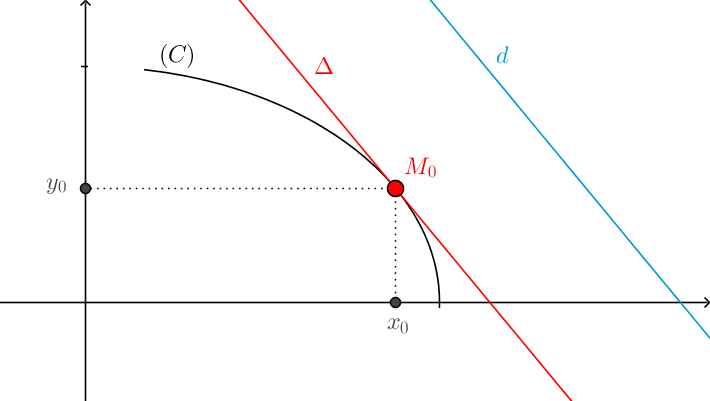### The equation of a tangent which is parallel to a given line

Thứ sáu - 31/01/2020 11:06
This article shows how to write the equation of a tangent which is parallel to a given line.
The following facts are regularly used.

 $(i)$ If $\left( d \right)$ has its equation of form $\left( d \right):y = kx + b$, then its slope is $k$. $(ii)$ The slope of the tangent $\Delta$ of the graph of function $y=f\left( x \right)$  at $x_0$ is $f'\left( {{x_0}} \right)$. $(iii)$ Every two parallel lines have the same slope. $(iv)$ The equation of the targent $\Delta$ of the fuction $y=f\left( x \right)$  at  $M_0(x_0;y_0)$ is $$y = f'\left( {{x_0}} \right)\left( {x - {x_0}} \right) + {y_0}.\;\;$$Problem. Write the equation of the tangent $(\Delta)$ of the graph $\left( C \right):y = f\left( x \right)$ paralleling to the strange line $\left( d \right):y = kx + b$.

Solution. Let $M_0(x_0;y_0)$ be the point of tangency. It follows from $(i)$ and $(ii)$ that the slope of  $\Delta$ and $d$  are $f'\left( {{x_0}} \right)$ and $k$ respectively.

Since $\Delta \parallel d$, the statement $(iii)$ implies that $f'\left( {{x_0}} \right) = k$, from which it follows that $x_0$ is a solution of the equation $f'\left( {{x}} \right) = k$.

Therefore, to find equation of the tangent $(\Delta)$, we can do the following steps.

 Step 1. Solve the equation $f'\left( {{x}} \right) = k$. Its solution $x_0$, say, is the $x$-coordinate of the point of tangency.  Step 2. Calculate ${y_0} := f\left( {{x_0}} \right)$ to obtain $M_0(x_0;y_0)$.  Step 3. Write the equation of the tangent of the graph $\left( C \right)$ with the point of tangency $M_0(x_0;y_0)$ according to $(iv)$.

Example 1. Write the equation of the tangent $\Delta$ of $(C): y = {x^2} - 2x - 1$ paralleling to $\left( d \right):y = 2x - 1$.Solution. Step 1. We have $f\left( x \right) = {x^2} - 2x - 1 \Rightarrow f'\left( x \right) = 2x - 2.$ The $x$-coordinate of the point of tangency is the solution of the equation $$f'\left( x \right) = 2 \Leftrightarrow 2x - 2 = 2 \Leftrightarrow x = 2.$$
Step 2. Substitute $x_0=2$ into $(C)$ to obtain $y_0=-1$. It follows that the coordinate of the point of tangency is ${M_0}\left( {2; - 1} \right).$

Step 3. We also have $f'\left( {{x_0}} \right) = 2$. The equation of the tangent at ${M_0}\left( {2; - 1} \right)$  is
$$y = f'\left( {{x_0}} \right)\left( {x - {x_0}} \right) + {y_0} \Leftrightarrow y = 2\left( {x - 2} \right) - 1 \Leftrightarrow y = 2x - 5.$$

Example 2. Write the equaion of the tangent $\Delta$ of $\left( C \right): y = {x^3} + 3x - 1$ paralleling to $\left( d \right):y = 6x - 1$.

Solution. The $x$-coordinate of the point of tangency is the solution of the following equation.

$$f'\left( x \right) = 6 \Leftrightarrow 3{x^2} + 3 = 6 \Leftrightarrow \left[ \begin{array}{l} {x_1} = 1 \Rightarrow {y_1} = f\left( 1 \right) = 3\\ {x_2} = - 1 \Rightarrow {y_2} = f\left( 1 \right) = - 5 \end{array} \right.$$
Since there are two solutions, there exist two points of tangency ${M_1}\left( {1;3} \right),{M_2}\left( { - 1; - 5} \right)$.
The equation of the tangent at ${M_1}\left( {1;3} \right)$ is $$\left( {{\Delta_1}} \right):\;\;\;\;y = 6\left( {x - {x_1}} \right) + {y_1} \Leftrightarrow y = 6\left( {x - 1} \right) + 3 \Leftrightarrow y = 6x - 3.$$
Similarly, the equation of the tangent at ${M_2}\left( { - 1; - 5} \right)$ is $$\left( {{\Delta_2}} \right):\;\;\;\;y = 6\left( {x - {x_2}} \right) + {y_2} \Leftrightarrow y = 6\left( {x + 1} \right) - 5 \Leftrightarrow y = 6x + 1.$$

Tổng số điểm của bài viết là: 5 trong 1 đánh giá

Xếp hạng: 5 - 1 phiếu bầu
Click để đánh giá bài viết

Ý kiến bạn đọc

Chương trình